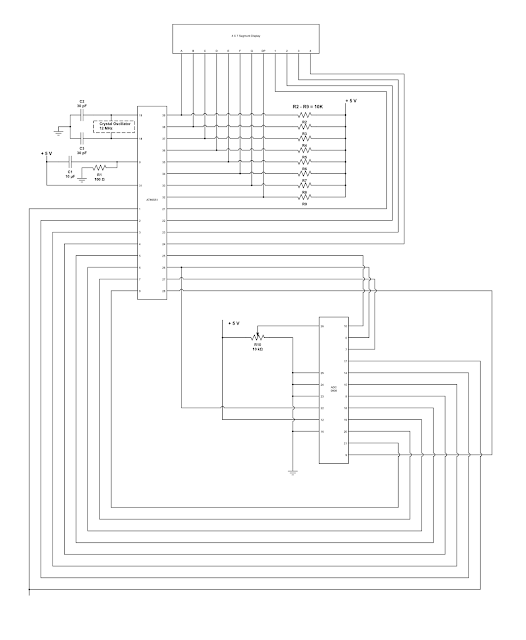### Digital Voltmeter using AT89S51 microcontroller

This project is designed to get the voltage value to be display on the digital display. In this project we used AT89S51 microcontroller to control and generate the signals. ADC0808 converts the analog voltage value into digital value. This digital value is applied to the controller and controller will generate the signals to show the digital value on the display.

Here as you can see the circuit measures the value of the voltage across variable resistor R10. As we change the resistance of the pot, the voltage across it also changes. Therefore the change on display also takes place. If we want to measure the analog voltage across two points then connect pin no. 26 of ADC 0808 to one point and another point to ground.Circuit Diagram

Pin no. 25 to 28 are the address pins to select the analog input. Since the pins are connected to ground ADC will convert the analog input at IN0 pin (pin no. 26). The binary value of analog voltage is generated at the output of the ADC 0808.

To download the proteus simulation file and codes for this project click on below button

Previous
Next Post »